# transformTransform属性应用于元素的2D或3D转换。这个属性允许你将元素旋转，缩放，移动，倾斜等。

CSS3 transform 属性

Transform属性应用于元素的2D或3D转换。这个属性允许你将元素旋转，缩放，移动，倾斜等。

 默认值： none 继承： no 版本： CSS3 JavaScript 语法： object.style.transform="rotate(7deg)"

 属性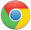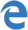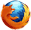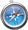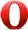transform (2D) 36.04.0 -webkit- 10.09.0 -ms- 16.03.5 -moz- 9.03.2 -webkit- 23.015.0 -webkit-10.5 -o- transform (3D) 36.012.0 -webkit- 12.0 10.0 16.010.0 -moz- 9.04.0 -webkit-

`transform: none|transform-functions;`
 值 描述 none 定义不进行转换。 matrix(n,n,n,n,n,n) 定义 2D 转换，使用六个值的矩阵。 matrix3d(n,n,n,n,n,n,n,n,n,n,n,n,n,n,n,n) 定义 3D 转换，使用 16 个值的 4x4 矩阵。 translate(x,y) 定义 2D 转换。 translate3d(x,y,z) 定义 3D 转换。 translateX(x) 定义转换，只是用 X 轴的值。 translateY(y) 定义转换，只是用 Y 轴的值。 translateZ(z) 定义 3D 转换，只是用 Z 轴的值。 scale(x[,y]?) 定义 2D 缩放转换。 scale3d(x,y,z) 定义 3D 缩放转换。 scaleX(x) 通过设置 X 轴的值来定义缩放转换。 scaleY(y) 通过设置 Y 轴的值来定义缩放转换。 scaleZ(z) 通过设置 Z 轴的值来定义 3D 缩放转换。 rotate(angle) 定义 2D 旋转，在参数中规定角度。 rotate3d(x,y,z,angle) 定义 3D 旋转。 rotateX(angle) 定义沿着 X 轴的 3D 旋转。 rotateY(angle) 定义沿着 Y 轴的 3D 旋转。 rotateZ(angle) 定义沿着 Z 轴的 3D 旋转。 skew(x-angle,y-angle) 定义沿着 X 和 Y 轴的 2D 倾斜转换。 skewX(angle) 定义沿着 X 轴的 2D 倾斜转换。 skewY(angle) 定义沿着 Y 轴的 2D 倾斜转换。 perspective(n) 为 3D 转换元素定义透视视图。

## 实例

```div
{
transform:rotate(7deg);
-ms-transform:rotate(7deg); /* IE 9 */
-webkit-transform:rotate(7deg); /* Safari and Chrome */
}```

php中文网
phpstudy

QQ交流群